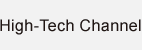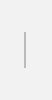#The world of units has international standards. SI is an abbreviation of “Le Système International d'Unités”, which is French for “system of international units”. At the end of the 18th century, there were growing calls to use units that exceeded international boundaries. First, the “Treaty of the Metre” was adopted in 1875. This was still unable to be unified in some fields, so SI units were adopted as practical units at the 11th General Conference on Weights and Measures in 1960.

The below “seven SI base units” were established as the SI units system.

## SI base units

• Metre (length)
• Kilogram (mass)
• Second (time)
• Ampere (electric current)
• Kelvin (thermodynamic temperature)
• Mole (amount of substance)
• Candela (luminous intensity)

There are also derived units such as square metres (area), cubic metres (volume), metres per second (acceleration), and radian (plane angle).

There are also “SI prefixes”, which are symbols that express multiples and quantities. You will probably be familiar with “centi-”, “milli-”, “mega- “and “giga-“. Adding words to a unit to express, for example, 10-times, 100-times, 1,000-time or one-tenth, one-hundredth or one-thousandth, enables us to express large and small figures.

There are also some non-SI units that are accepted for use with the SI (hereinafter referred to as “accepted non-SI units”). These include litres, tons, hectares, minutes, hours, and days.